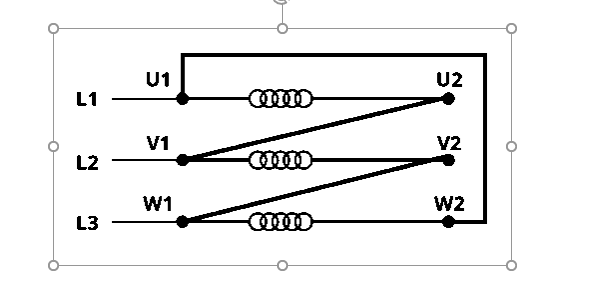## Star Delta Starter, motor connection, control circuit, working principle...DeltaStar
Star Delta Starter is a very common type of starter and widely used compared to other type of induction motor starting method. A star delta is mainly used for a cage motor (M) designed to run normally on a delta connected stator (S) winding.

Star delta starter

When the switch S is in the START position, the stator windings are connected to the star.

When the engine picks up speed, about 80 percent of its rated speed, the S switch is immediately put into the RUN position. As a result, the stator coil that was in a star connection has now been changed to a DELTA connection.

the stator winding of the Star delta starter is connected to the star and then to the delta so that the starting line current of the motor is reduced to 1/3 compared to the starting current (I) with the windings connected in the delta. when the stator (S) windings are star connected, each stator (S) phase gets a voltage of VL/√3. Here VL is the line voltage.

Since the developing torque is proportional to the square of the voltage applied to an induction motor. Star delta starter reduces the starting torque to one-third that obtained by direct delta starting.

### Star Delta Starter Theory With Induction Motor Starting Method

At the start of an induction motor, the stator windings are star connected, therefore, the voltage across each phase winding is 1 / √3 times the line voltage.

VL is the line voltage,

Istyp is the starting current of each phase with the stator windings connected to the star,

Istyl : starting line current (I) with the stator (S) winding in the star.

For a star connection, the line current is equal to the phase current.

V1 is the phase voltage,

VL is the line voltage of Star delta starter,

IstΔp: starting current (I) of each phase by switching with the stator (S) windings connected in delta,

IstΔl: starting line current (I) by switching with the stator (S) windings in delta,

IscΔp: short circuit phase current (I) by switching with the stator (S) windings in delta,

Z10 is the full-stop equivalent impedance for each phase of the motor, referred to as the stator.

For a delta connection, the line current is equal to root three times the phase current.

with a star delta starter, the starting current from the main supply is one third of the direct delta switching current.

with star-delta starting, the initial torque is reduced to one-third of the torque obtained through direct-delta switching.

IflΔp: full charge or load phase intensity with the coil in delta

Related topics:

Star delta starter;

Star delta connection;

Star and delta connection.

star-delta motor connection

Star delta transformation

Star-Delta Starter components selection

Star delta control circuit

star delta starter control wiring diagram with timer

star delta starter working principle

automatic star delta starter diagram